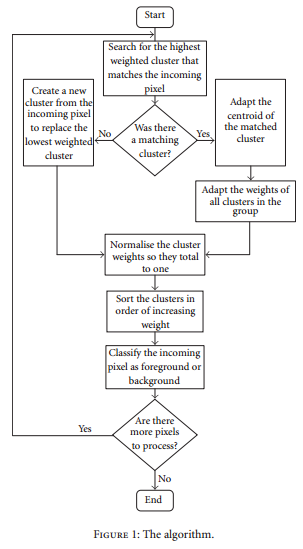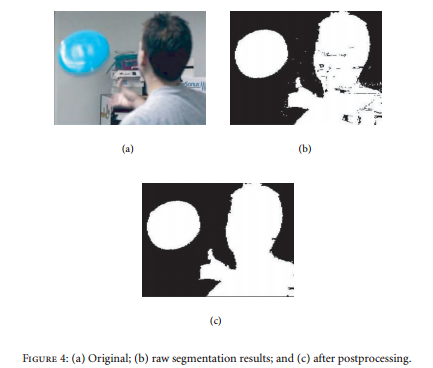K-means is a typical clustering method, which can also be used for motion detection.

### Steps

1. For each pixel in the video, based on its previous values, a group of $$K$$ (usually $$K$$ = 3~5) clusters are clustered by using K-means. For each cluster, a weight $$w_k$$ (the more samples it contains, the higher the weight is) and a center $$c_k$$ (mean) are stored.

2. For each new frame, the new pixel value is compared with each cluster of the pixel (the Manhattan distance between the new pixel value and the center of each cluster is calculated). a. If no cluster matches, (i.e. all distances are bigger than a threshold), the cluster with the lowest weight will be replaced by a new cluster with its mean equal to the new pixel value (similar to GMM). b. Else if a cluster matches, (i.e. A: the distance between its center and the new pixel value is smaller than a threshold, and at the same time B: the cluster has the highest weight among the clusters that satisfy condition A), the weight and the center of that cluster are updated.

3. Normalize the weights for all the clusters.

4. Sort all the clusters from high to low according to their weights.

5. Sum all the weights of the clusters which are before the cluster that the new pixel belongs to. The higher the sum is, the higher probability the new pixel belongs to a foreground, vise versa. $$P = \sum^{K-1}_{k>M_k}w_k$$,

where $$M_k$$ is the cluster index of the matching cluster.

The pipeline of the K-means for motion detection is shown in Fig. 1.The authors also use a connected components algorithm for the post-processing. Example results are shown in Fig. 2.